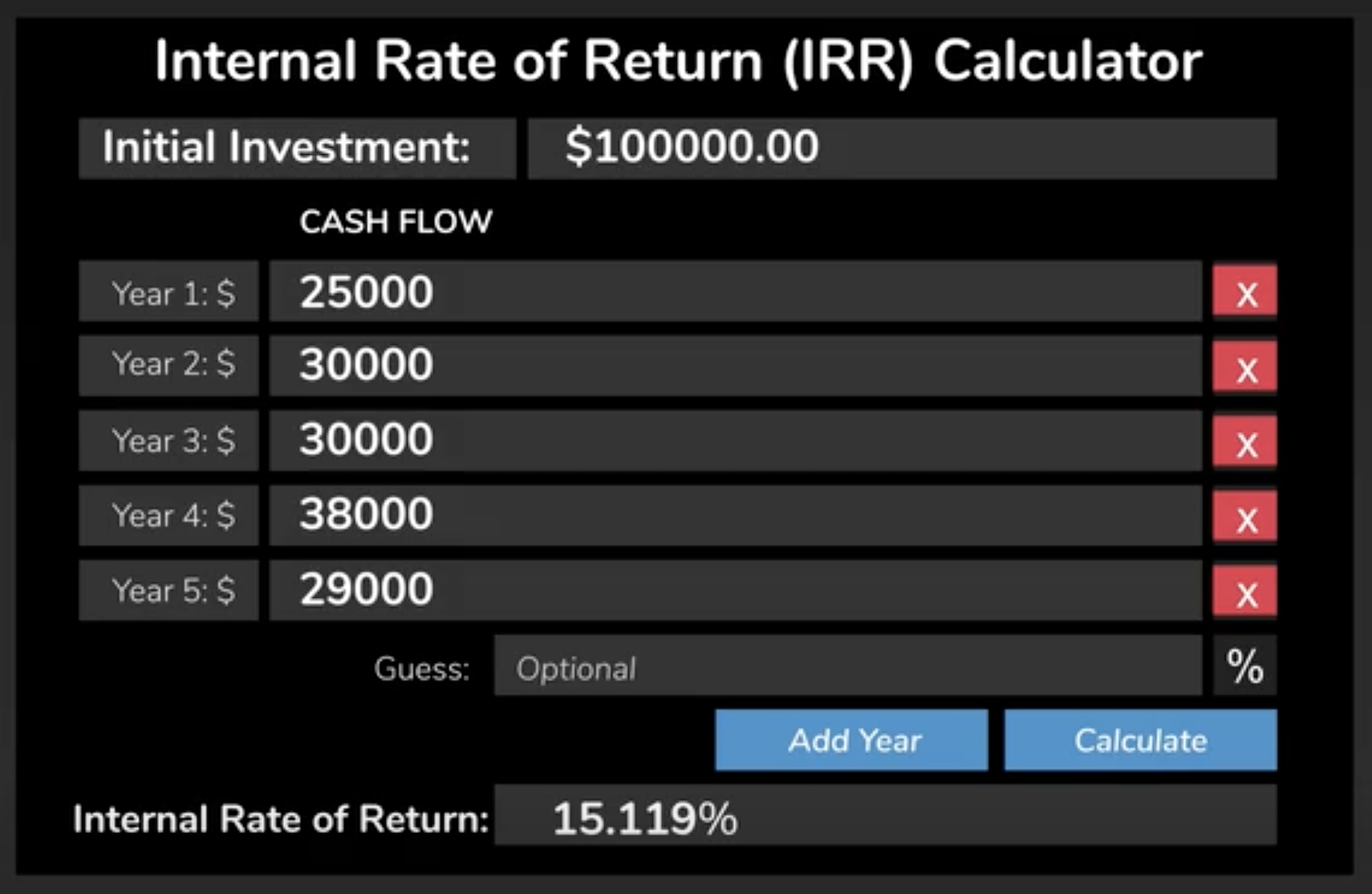+

# Internal Rate of Return

Author: Sophia Tutorial
##### Description:

Identify the advantages and disadvantages of IRR as a capital budget method.

(more)### Developing Effective Teams

*No strings attached. This college course is 100% free and is worth 1 semester credit.

37 Sophia partners guarantee credit transfer.

299 Institutions have accepted or given pre-approval for credit transfer.

* The American Council on Education's College Credit Recommendation Service (ACE Credit®) has evaluated and recommended college credit for 33 of Sophia’s online courses. Many different colleges and universities consider ACE CREDIT recommendations in determining the applicability to their course and degree programs.

Tutorial

what's covered
In this lesson, you will learn about the advantages and disadvantages of the internal rate of return as a capital budget method. Specifically, this lesson will cover:
1. Defining the Internal Rate of Return

## 1. Defining the Internal Rate of Return

As mentioned in an earlier lesson, the internal rate of return (IRR) is an investment evaluation method that produces the discount rate at which the net present value of cost equals the net present value of the benefits.

The higher the project's IRR, the more desirable it is to undertake the project. A firm should, in theory, take on all the available projects that exceed its cost of capital; however, investments may limit the availability of funds and so projects have to be ranked.

After we have analyzed the cash flows (both revenues and expenses) and know the time that the project life will cover, we could calculate the internal rate of return using an app or spreadsheet software.

### IN CONTEXT

A web app was used to show the following initial investment of a \$100,000 project. Notice \$100,000 was initially spent, which we can consider as Year 0. In Year 1, the project earned \$25,000, in Year 2, it earned \$30,000, and so on.

What is the discount rate that makes the present value of the inflows from Year 1 through 5 equal to the initial investment of \$100,000?Using software, the internal rate of return (IRR), is found to be 15.119%. This project should be accepted if 15.119% is greater than the cost of capital.
term to know

The Internal Rate of Return (IRR)
The discount rate at which the net present value of costs (negative cash flows) of the investment equals the net present value of the benefits (positive cash flows) of the investment.

The IRR has some advantages to its methodology.

• It is clear and easy to understand as a rate.
• It is easy to compare to the threshold rate of return
• It recognizes the time value of money in value and cash flows.
There also disadvantages to the internal rate of return.
• It can overstate the rate of return for a project that assumes cash flows are not reinvested at a rate lower than the calculated internal rate of return. This reflects a reinvestment rate risk.
• It does not consider the cost of capital; therefore, it should not be used to compare projects of different durations.
• It may have multiple values in the case of positive cash flows, followed by negative cash flows, and then again by positive cash flows
In a situation where a company is considering mutually exclusive projects (meaning that they will only do one of a set of projects), sometimes the IRR can rank one project ahead of the other while the other has a higher net present value. When this happens, which one should be selected?

In this case, the one with the higher net present value should be selected because the business wants a higher dollar return, not necessarily a higher interest rate.

summary
In this lesson, you learned that the internal rate of return, or IRR, is one of the project investment tools that produces the discount rate or the net present value when the cost of the investment equals zero. The higher the internal rate of return, the more desirable a project is. It can be calculated using spreadsheet software or a web app.

Advantages of the IRR include the fact that it is clear and easy to understand, uses cash flows that recognize the time value of money, and can be easily compared to the company's minimum acceptable rate of return.

There are also disadvantages. It can overstate the rate of return for a project using cash flows that can't be invested at the calculated IRR. It does not consider the cost of capital, so it should not be used to compare projects of different durations. And finally, it should not be used to evaluate mutually exclusive projects, because it could present a favorable rate of return for one project when the economic value of another project is actually greater.

Best of luck in your learning!

Source: THIS CONTENT HAS BEEN ADAPTED FROM LUMEN LEARNING'S “Internal Rate of Return” TUTORIAL.

Terms to Know
Internal Rate of Return (IRR)

The discount rate at which the net present value of costs (negative cash flows) of the investment equals the net present value of the benefits (positive cash flows) of the investment.

Rating